Case Based Questions (MCQ)

Chapter 5 Class 12 Continuity and Differentiability
Serial order wise

## Based on the above information, answer any four  of the following questions.  Let f(x) = sin x and g(x) = x3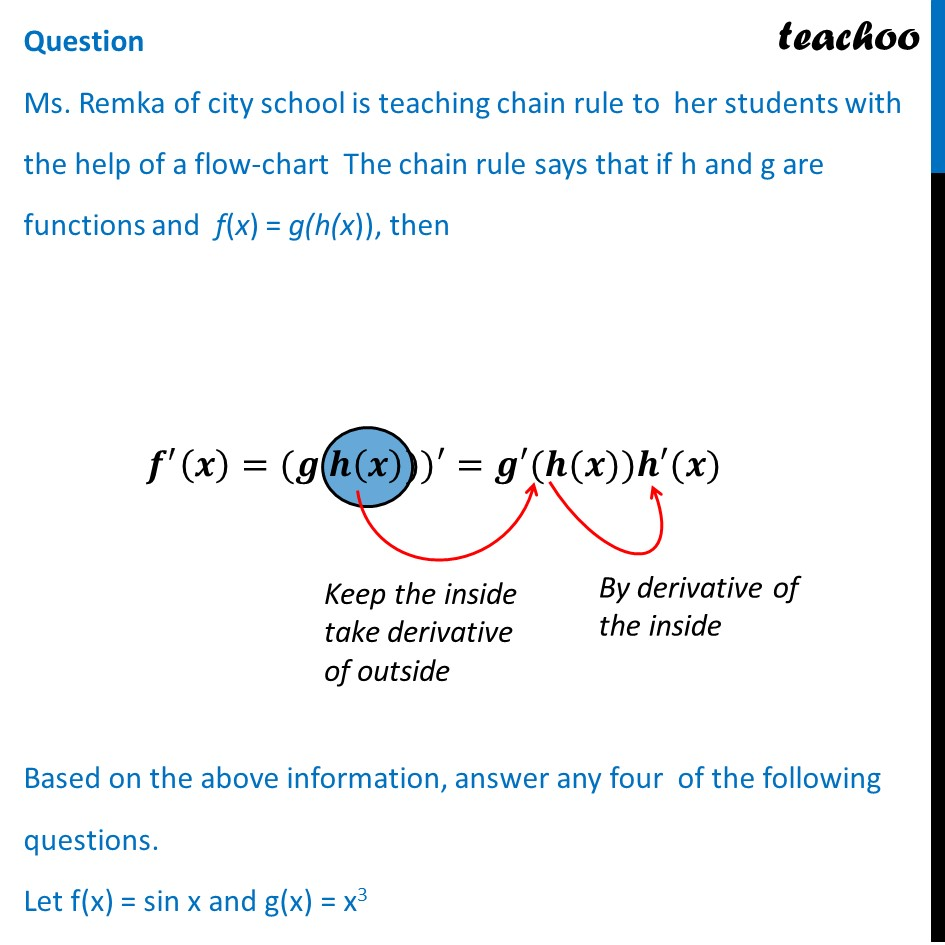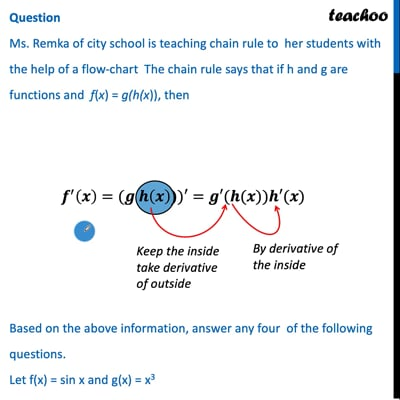This video is only available for Teachoo black users

## (d) 3 sin x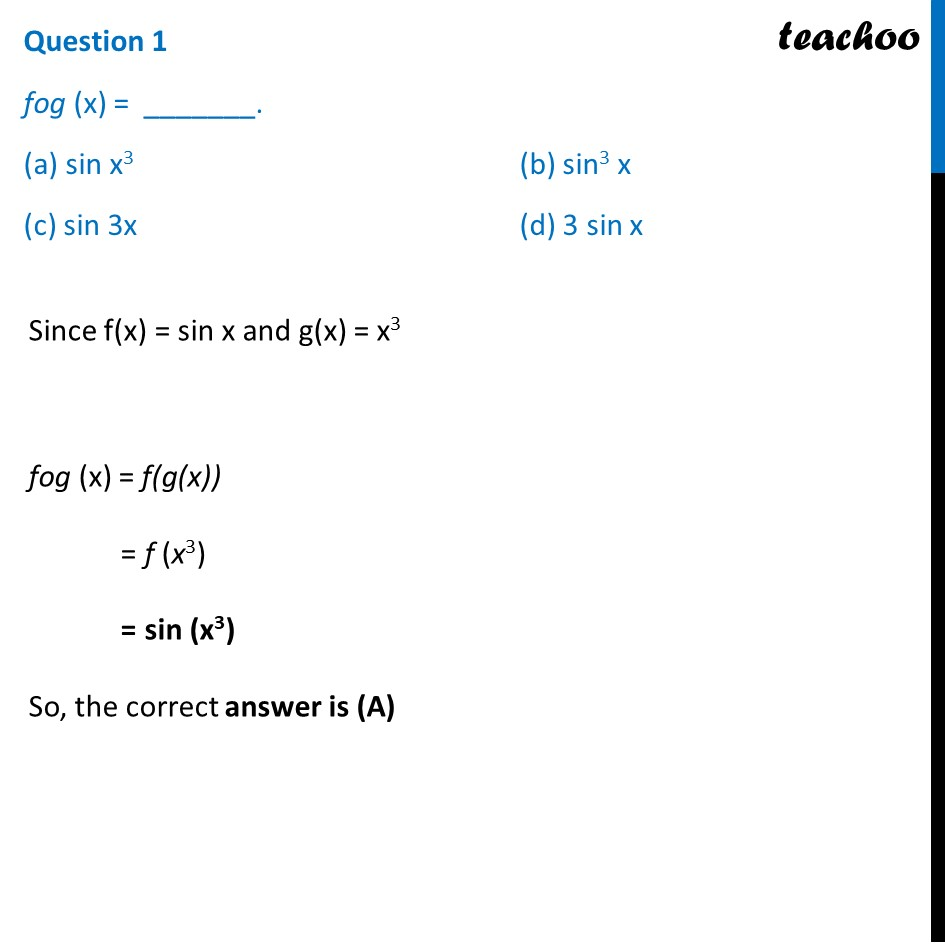## (d) 3 sin x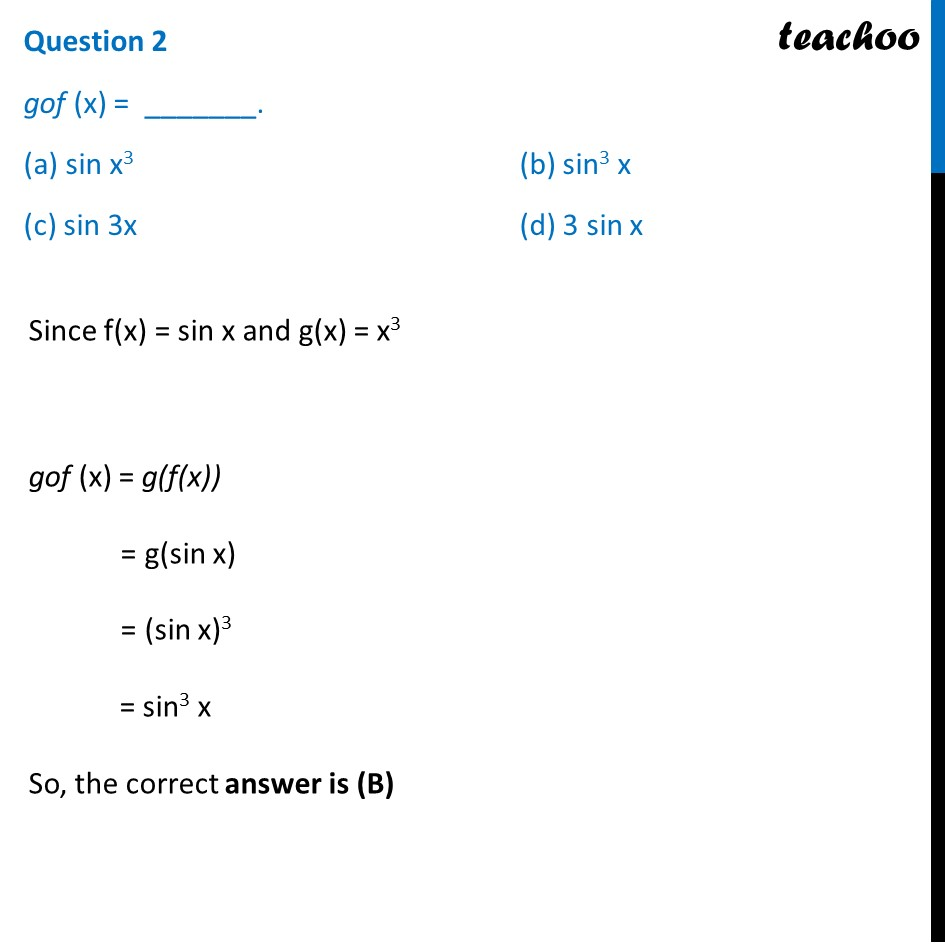## (d) −cos3 x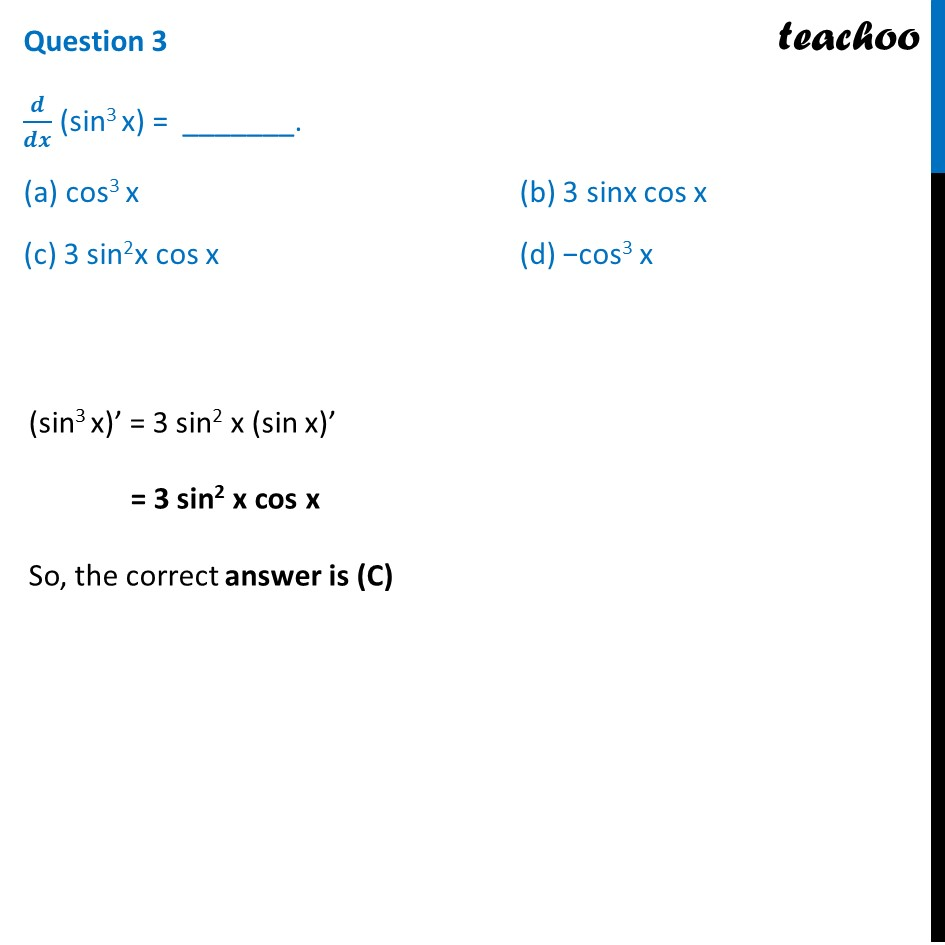## (d) 3x2 cos (x3)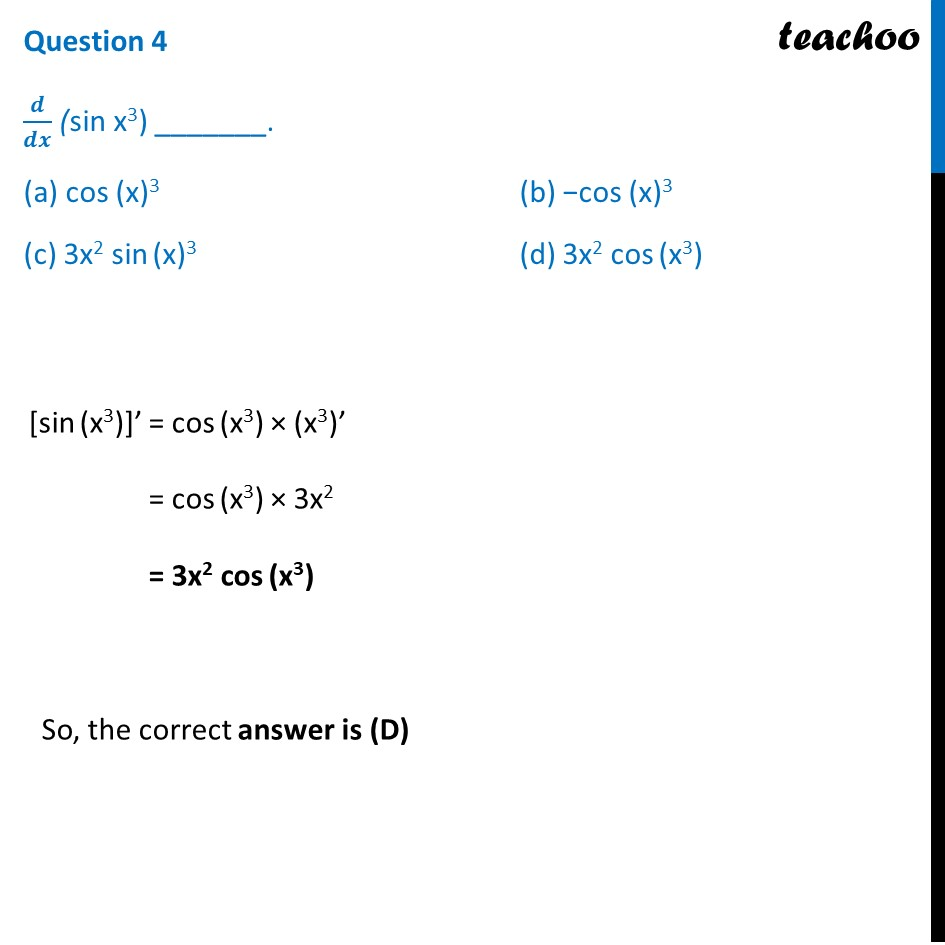## (d) −2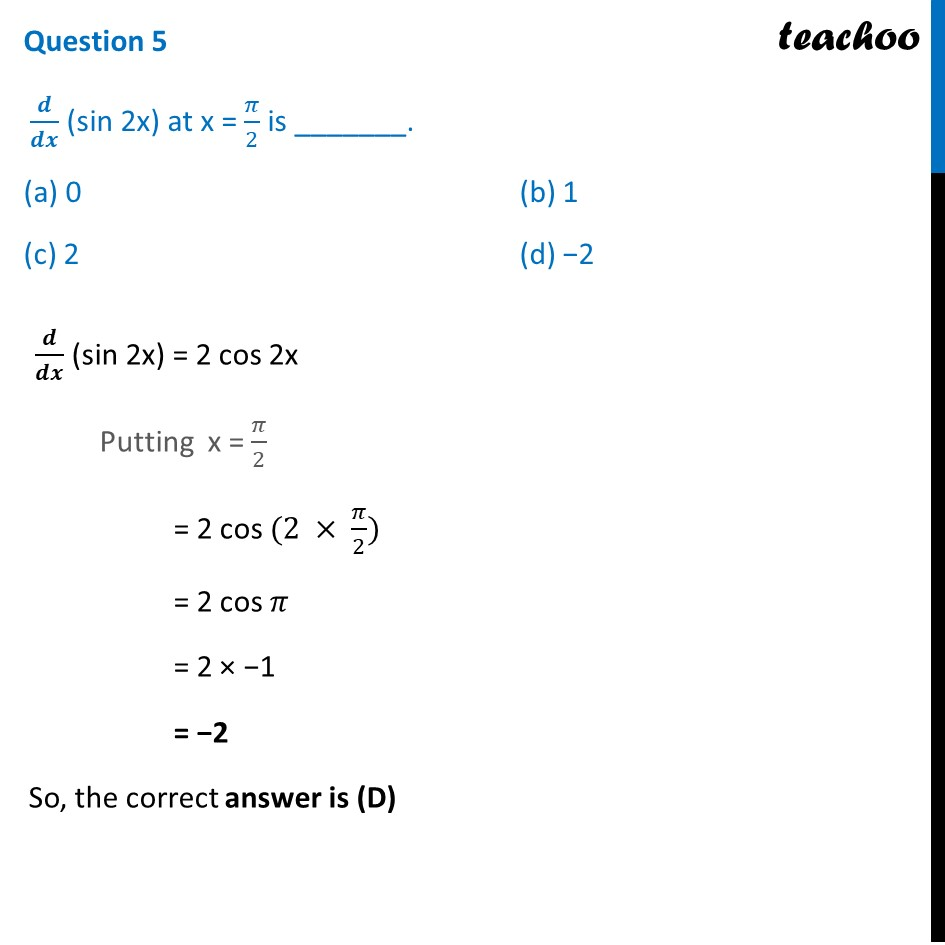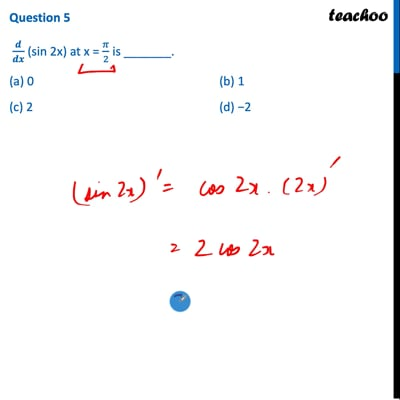This video is only available for Teachoo black users

Introducing your new favourite teacher - Teachoo Black, at only ₹83 per month

### Transcript

Question Ms. Remka of city school is teaching chain rule to her students with the help of a flow-chart The chain rule says that if h and g are functions and f(x) = g(h(x)), then Based on the above information, answer any four of the following questions. Let f(x) = sin x and g(x) = x3 Question 1 fog (x) = _______. (a) sin x3 (b) sin3 x (c) sin 3x (d) 3 sin x Since f(x) = sin x and g(x) = x3 fog (x) = f(g(x)) = f (x3) = sin (x3) So, the correct answer is (A) Question 2 gof (x) = _______. (a) sin x3 (b) sin3 x (c) sin 3x (d) 3 sin x Since f(x) = sin x and g(x) = x3 gof (x) = g(f(x)) = g(sin x) = (sin x)3 = sin3 x So, the correct answer is (B) Question 3 𝒅/𝒅𝒙 (sin3 x) = _______. (a) cos3 x (b) 3 sinx cos x (c) 3 sin2x cos x (d) −cos3 x (sin3 x)’ = 3 sin2 x (sin x)’ = 3 sin2 x cos x So, the correct answer is (C) Question 4 𝒅/𝒅𝒙 (sin x3) _______. (a) cos (x)3 (b) −cos (x)3 (c) 3x2 sin (x)3 (d) 3x2 cos (x3) [sin (x3)]’ = cos (x3) × (x3)’ = cos (x3) × 3x2 = 3x2 cos (x3) So, the correct answer is (D) Question 5 𝒅/𝒅𝒙 (sin 2x) at x = 𝜋/2 is _______. (a) 0 (b) 1 (c) 2 (d) −2 𝒅/𝒅𝒙 (sin 2x) = 2 cos 2x Putting x = 𝜋/2 = 2 cos (2 × 𝜋/2) = 2 cos 𝜋 = 2 × −1 = −2 So, the correct answer is (D)